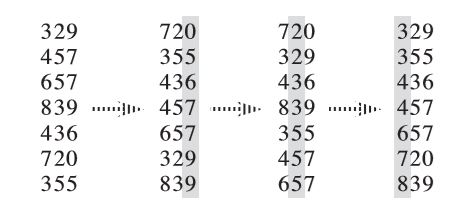# 基数排序

## 过程## 算法实现

### 伪代码### C++

  1 2 3 4 5 6 7 8 9 10 11 12 13 14 15 16 17 18 19 20 21 22 23 24 25 26 27 28 29 30 31 32 33 34 35 const int N = 100010; const int W = 100010; const int K = 100; int n, w[K], k, cnt[W]; struct Element { int key[K]; bool operator<(const Element& y) const { // 两个元素的比较流程 for (int i = 1; i <= k; ++i) { if (key[i] == y.key[i]) continue; return key[i] < y.key[i]; } return false; } } a[N], b[N]; void counting_sort(int p) { memset(cnt, 0, sizeof(cnt)); for (int i = 1; i <= n; ++i) ++cnt[a[i].key[p]]; for (int i = 1; i <= w[p]; ++i) cnt[i] += cnt[i - 1]; // 为保证排序的稳定性，此处循环i应从n到1 // 即当两元素关键字的值相同时，原先排在后面的元素在排序后仍应排在后面 for (int i = n; i >= 1; --i) b[cnt[a[i].key[p]]--] = a[i]; memcpy(a, b, sizeof(a)); } void radix_sort() { for (int i = k; i >= 1; --i) { // 借助计数排序完成对关键字的排序 counting_sort(i); } } 

  1 2 3 4 5 6 7 8 9 10 11 12 13 14 15 16 17 18 19 20 21 22 23 24 25 26 27 28 29 30 31 32 33 34 35 #include #include #include void radix_sort(int n, int a[]) { int *b = new int[n]; // 临时空间 int *cnt = new int[1 << 8]; int mask = (1 << 8) - 1; int *x = a, *y = b; for (int i = 0; i < 32; i += 8) { for (int j = 0; j != (1 << 8); ++j) cnt[j] = 0; for (int j = 0; j != n; ++j) ++cnt[x[j] >> i & mask]; for (int sum = 0, j = 0; j != (1 << 8); ++j) { // 等价于 std::exclusive_scan(cnt, cnt + (1 << 8), cnt, 0); sum += cnt[j], cnt[j] = sum - cnt[j]; } for (int j = 0; j != n; ++j) y[cnt[x[j] >> i & mask]++] = x[j]; std::swap(x, y); } delete[] cnt; delete[] b; } int main() { std::ios::sync_with_stdio(false); std::cin.tie(0); int n; std::cin >> n; int *a = new int[n]; for (int i = 0; i < n; ++i) std::cin >> a[i]; radix_sort(n, a); for (int i = 0; i < n; ++i) std::cout << a[i] << ' '; delete[] a; return 0; } 

## 参考资料与注释

1. Thomas H. Cormen, Charles E. Leiserson, Ronald L. Rivest, and Clifford Stein.Introduction to Algorithms(3rd ed.). MIT Press and McGraw-Hill, 2009. ISBN 978-0-262-03384-8. "8.3 Radix sort", pp. 199.